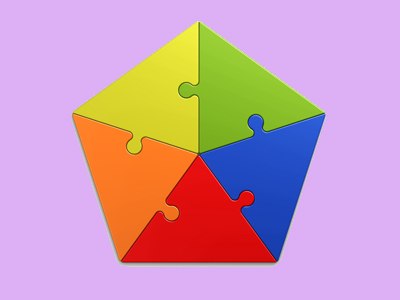A pentagon is not a quadrilateral.

# Shapes (Year 5)

In KS2 Maths as children advance to Year Five their knowledge of shapes will increase. By now they should know the names of the different 2D shapes from the circle to the decagon, and also many of the 3D shapes. They should also be able to recognise an equilateral, a right angle and an isosceles triangle and will now be taught about scalene and obtuse triangles and congruent shapes.

A circle and a triangle are both shapes. Did you know it is said that only a genius can draw a perfect circle? Have a go and see if you are a genius! There are various types of triangles, like Isosceles, scalene or equilateral, depending on the size of their angles.

Do you know the difference between an oblong and a rectangle? Can you tell an isosceles from a scalene triangle? See how much you remember about shapes by playing this quiz.

1.
What is the name given to the distance from one edge of a circle to the other through the centre?
Circumference
Tangent
Diameter
The diameter is twice the radius
2.
What is a triangle with no equal sides and no equal angles called?
Isosceles
Equilateral
Scalene
Right angled
Scalene comes from the Greek word for "unequal"
3.
What is the name given to polygons with all the sides and angles equal?
Irregular polygons
Regular polygons
Isosceles polygons
Equilateral polygons
Irregular polygons are where the sides are not equal
4.
Which of these shapes is not a quadrilateral?
Oblong
Rectangle
Pentagon
Square
Pentagons have 5 sides - all the other shapes have just 4
5.
What is the name given to a triangle with an angle larger than 90o?
Isosceles
Equilateral
Scalene
Obtuse
Do you remember that angles between 90o and 180o are called obtuse angles?
6.
What is an oblong?
A rectangle that is not a square
A rectangle that is a square
A quadrilateral that is not a square
A quadrilateral that is a square
A rectangle is a quadrilateral where all four sides are right angles
7.
How do we know if two triangles are congruent?
If they both have the same three sides
If they both have a right angle
If they both have the same size angles
If they both have two sides the same
If one shape can become identical to another by using turns, flips or slides but without changing the size, then the two shapes are congruent
8.
What is an octahedron?
3D shape with octagon faces
3D shape with 8 faces
2D shape with 8 sides
2D shape with 8 angles
Remember that 'octa' means 8 and that 'hedron' means a 3D shape
9.
What is the shape of the faces of a regular octahedron?
Square
Octagon
Hexagon
Triangle
A regular octahedron would have equilateral triangles as each of its 8 faces
10.
If you cut a triangular prism in half, what shape would the new face be?
Circle
Triangle
Square
Hexagon
If you cut a triangle into two with a straight line, at least one of the two pieces will be a triange

Author:  Amanda Swift